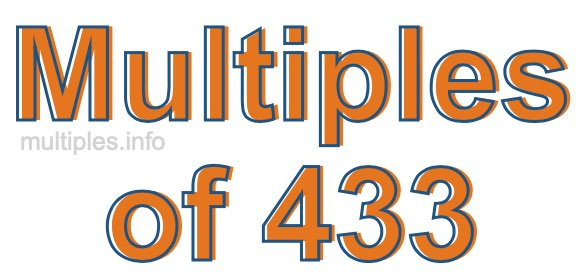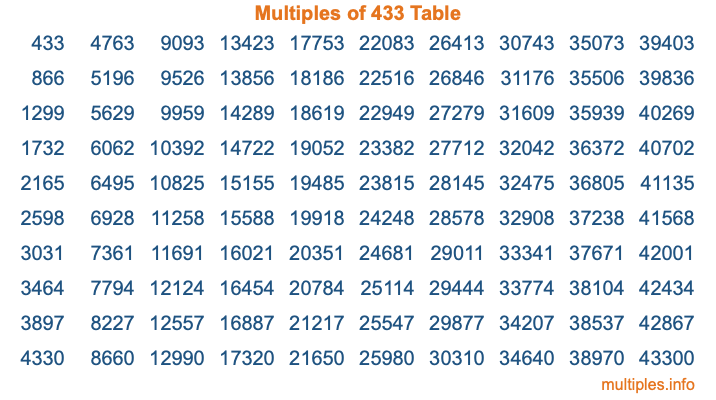Multiples of 433Welcome to the Multiples of 433 page. Here we will first teach you everything you will ever need to know about the multiples of 433, and then give you a study guide summary of everything we taught you to make sure you remember it all. Use this page to look up facts and learn information about the multiples of 433. This page will make you a multiples of four hundred thirty-three expert!

Definition of Multiples of 433
Multiples of 433 are all the numbers that when divided by 433 equal an integer. Each of the multiples of 433 are called a multiple. A multiple of 433 is created by multiplying 433 by an integer.

Therefore, to create a list of multiples of 433, you start with 1 multiplied by 433, then 2 multiplied by 433, then 3 multiplied by 433, and so on for as long as you want. Thus, the list of the first five multiples of 433 is 433, 866, 1299, 1732, and 2165. To see a larger list of multiples of 433, see the printable image of Multiples of 433 further down on this page. We also have a category where you can choose any nth multiple of 433.

Multiples of 433 Checker
The Multiples of 433 Checker below checks to see if any number of your choice is a multiple of 433. In other words, it checks to see if there is any number (integer) that when multiplied by 433 will equal your number. To do that, we divide your number by 433. If the the quotient is an integer, then your number is a multiple of 433.

Is  a multiple of 433?

Least Common Multiple of 433 and ...
A Least Common Multiple (LCM) is the lowest multiple that two or more numbers have in common. This is also called the smallest common multiple or lowest common multiple and is useful to know when you are adding our subtracting fractions. Enter one or more numbers below (433 is already entered) to find the LCM.

Check out our LCM Calculator if you need more details about the Least Common Multiple or if you need the LCM for different numbers for adding and subtraction fractions.

nth Multiple of 433
As we stated above, 433 is the first multiple of 433, 866 is the second multiple of 433, 1299 is the third multiple of 433, and so on. Enter a number below to find the nth multiple of 433.

th multiple of 433

Multiples of 433 vs Factors of 433
433 is a multiple of 433 and a factor of 433, but that is where the similarities end. All postive multiples of 433 are 433 or greater than 433. All positive factors of 433 are 433 or less than 433.

Below is the beginning list of multiples of 433 and the factors of 433 so you can compare:

Multiples of 433: 433, 866, 1299, 1732, 2165, etc.

Factors of 433: 1, 433

As you can see, the multiples of 433 are all the numbers that you can divide by 433 to get a whole number. The factors of 433, on the other hand, are all the whole numbers that you can multiply by another whole number to get 433.

It's also interesting to note that if a number (x) is a factor of 433, then 433 will also be a multiple of that number (x).

Multiples of 433 vs Divisors of 433
The divisors of 433 are all the integers that 433 can be divided by evenly. Below is a list of the divisors of 433.

Divisors of 433: 1, 433

The interesting thing to note here is that if you take any multiple of 433 and divide it by a divisor of 433, you will see that the quotient is an integer.

Multiples of 433 Table
Below is an image of the first 100 multiples of 433 in a table. The table is in chronological order, column by column. The first column has the first ten multiples of 433, the second column has the next ten multiples of 433, and so on.The Multiples of 433 Table is also referred to as the 433 Times Table or Times Table of 433. You are welcome to print out our table for your studies.

Negative Multiples of 433
Although not often discussed or needed in math, it is worth mentioning that you can make a list of negative multiples of 433 by multiplying 433 by -1, then by -2, then by -3, and so on, to get the following list of negative multiples of 433:

-433, -866, -1299, -1732, -2165, etc.

Multiples of 433 Summary
Below is a summary of important Multiples of 433 facts that we have discussed on this page. To retain the knowledge on this page, we recommend that you read through the summary and explain to yourself or a study partner why they hold true.

There are an infinite number of multiples of 433.

A multiple of 433 divided by 433 will equal a whole number.

433 divided by a factor of 433 equals a divisor of 433.

The nth multiple of 433 is n times 433.

The largest factor of 433 is equal to the first positive multiple of 433.

433 is a multiple of every factor of 433.

433 is a multiple of 433.

A multiple of 433 divided by a divisor of 433 equals an integer.

433 divided by a divisor of 433 equals a factor of 433.

Any integer times 433 will equal a multiple of 433.

Multiples of a Number
Here you can get the multiples of another number, all with the same attention to detail as we did for multiples of 433 on this page.

Multiples of
Multiples of 434
Did you find our page about multiples of four hundred thirty-three educational? Do you want more knowledge? Check out the multiples of the next number on our list!

Copyright  |   Privacy Policy  |   Disclaimer  |   Contact